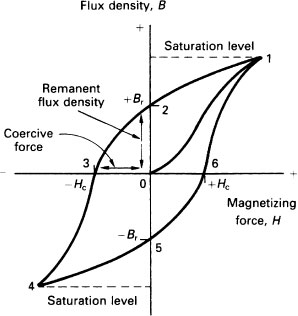16  Analogue Recording and Reproduction

Formulae

Magnetomotive force, m.m.f is given by

m.m.f. = NI

where N is the number of coil turns and I is the current.

The units of m.m.f are ampere turns/meter (At/m)

The intensity or field strength is the total force acting per unit length I of the magnetic circuit. It is called the magnetizing force H.

H = m.m.f./l = NI/l(AT/m)

The magnetizing force causes a flux Ф in the magnetic circuit.

The flux density B is the flux per unit area. The unit is the tesla (= webers/m2).

Ф = m.m.f./S

where S is the reluctance of the circuit.

Figure 73 shows a typical BH loop (hysteresis loop).Figure 73  Hysteresis loop ...

Get Sound Engineer's Pocket Book, 2nd Edition now with the O’Reilly learning platform.

O’Reilly members experience books, live events, courses curated by job role, and more from O’Reilly and nearly 200 top publishers.